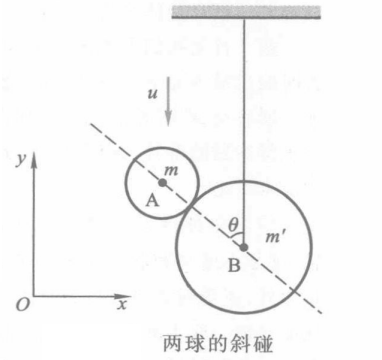$$m v_x+m^{\prime} v^{\prime}=0$$

$$\begin{gathered} m v_x=-F \sin \theta \Delta t \\ m v_y-(-m u)=F \cos \theta \Delta t \end{gathered}$$

$$\frac{v_y+u}{v_x}=-\cot \theta$$$$e=\frac{-\left(v^{\prime}-v_x\right) \sin \theta-v_y \cos \theta}{-u \cos \theta}$$

\begin{aligned} & v_x=-u \frac{m^{\prime}(1+e) \sin \theta \cos \theta}{m^{\prime}+m \sin ^2 \theta} \\ & v_y=-u\left[1-\frac{m^{\prime}(1+e) \cos ^2 \theta}{m^{\prime}+m \sin ^2 \theta}\right] \end{aligned}

①点击 收藏 此题， 扫码注册关注公众号接收信息推送（一月四份试卷,中1+高2+研1）
② 程序开发、服务器资源都需要大量的钱，如果你感觉本站好或者受到到帮助，欢迎赞助本站,赞助方式：微信/支付宝转账到 18155261033

 ①此题难易度如何 ②此题推荐度如何 确定